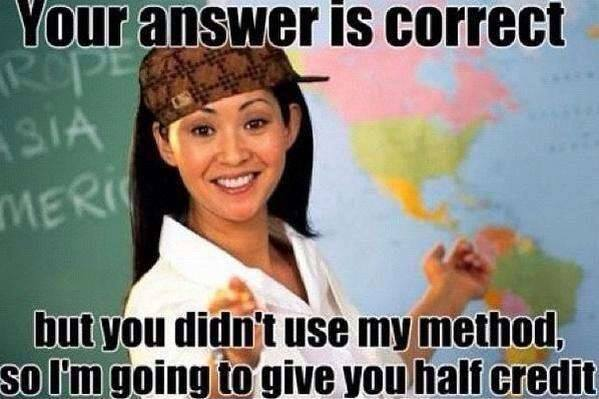# Your answer is correct, but you didn't use the correct method, so I am going to give you half credit

Algebra Level 1Tom was unhappy because he didn't get a full credit for this question:

Simplify $\frac{16}{64}$.

"Teacher, why I can't get full credit for this question?"

"Your answer is correct, but you didn't use the correct method, so I am going to give you half credit."

Actually what Tom did was simply cancel the two "6" that appear in the question as shown: $\frac{\overline{ab}}{\overline{bc}}=\frac{a}{c}$ $\frac{16}{64}=\frac{1\boxed{6}}{\boxed{6}4}=\frac{1}{4}$ Although Tom had gotten the correct answer, his method was wrong since 16 and 64 are 10+6 and 60+4 but not 1x6 and 6x4. Therefore, the "6" cannot be cancelled as shown in this method. But sometimes Tom's method is lucky and computes the correct solution.

For which of these fractions will Tom's method give a correct answer?

×